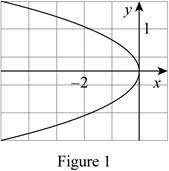Chapter 10.5, Problem 9E

Chapter
Section
Textbook Problem

# Find an equation of the parabola. Then find the focus and directrix.9.To determine

To Find: The equation of the parabola, focus and the directrix by using figure (1).Explanation

Given:

By referring the figure (1), we can find the value of vertex and point of the parabola,

Then the vertex is (0,0) and point of the parabola is (1,1) respectively.

Calculation:

By referring the figure (1) equation is to be,

y2=4px (1)

Substitute the value 1 for x and 1 for y in the equation (1).

12=4p×(1)1=4pp=14

Then, the focus of the graph is to be (p,0) .

Therefore, the focus of the parabola is (14,0)_

Compute the directrix of the parabola

### Still sussing out bartleby?

Check out a sample textbook solution.

See a sample solution

#### The Solution to Your Study Problems

Bartleby provides explanations to thousands of textbook problems written by our experts, many with advanced degrees!

Get Started

#### Sketch the graphs of the equations in Exercises 512. yx=1

Finite Mathematics and Applied Calculus (MindTap Course List)

#### Calculate y'. 44. y=sinmxx

Single Variable Calculus: Early Transcendentals, Volume I

#### Solve

Mathematical Applications for the Management, Life, and Social Sciences

#### True or False: is a convergent series.

Study Guide for Stewart's Single Variable Calculus: Early Transcendentals, 8th